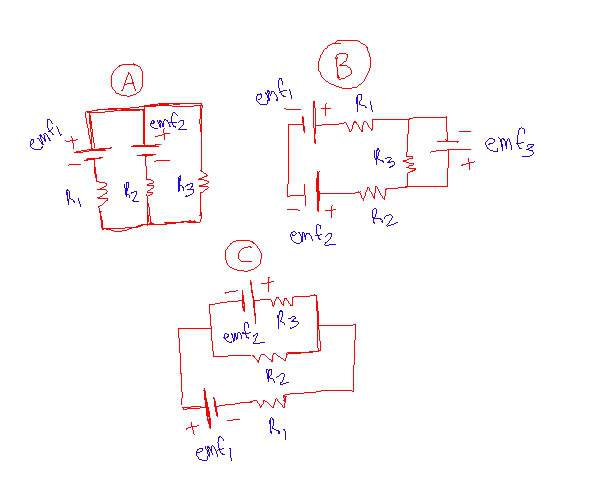# Circuits and Loop Rule

## Homework Statement

Write the loop rule for each of the following circuits:## Homework Equations

Depending on the loop:
-emf + IR1 + IR2 + .... + IRn = 0
V = IR
If in series: R1 + R2 + ... Rn = R
If in parallel: 1/R1 + 1/R2 + ... 1/Rn = 1/R

## The Attempt at a Solution

I really don't know how to do these because we usually only had 1 battery. But here's my shot:

(A) Could you say that both batteries are in series and treat them as one battery, then say the R2 and R3 are in parallel?

(B) and (C) I don't have the slightest clue. For (B), I don't know if you can say that R1, R2, and R3 are in series with emf3 there, and (C) we've never had a battery in parallel (We either had 1 battery or 2 batteries in series).

tiny-tim
Homework Helper
Write the loop rule for each of the following circuits:

(A) Could you say that both batteries are in series and treat them as one battery, then say the R2 and R3 are in parallel? …

Hi DeadFishFactory!"Loop rule" means that you only consider one loop at a time … that means everything is in one (series) circuit.

Forget the series and parallel rules … only apply Kirchhoff's rules …

voltage gain across each battery is the voltage (obviously!), and voltage drop across each resistor is I times R, and for each loop t all has to add to zero …

what do you get?(and don't forget to draw the currents in … I1 I2 I3 etc)